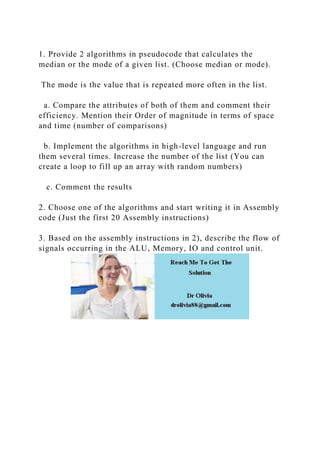Diese Präsentation wurde erfolgreich gemeldet.

# 1. Provide 2 algorithms in pseudocode that calculates the median or .docx

Anzeige
Anzeige
Anzeige
Anzeige
Anzeige
Anzeige
Anzeige
Anzeige
Anzeige
Anzeige
Anzeige×

1 von 1 Anzeige

# 1. Provide 2 algorithms in pseudocode that calculates the median or .docx

1. Provide 2 algorithms in pseudocode that calculates the median or the mode of a given list. (Choose median or mode).

The mode is the value that is repeated more often in the list.

a. Compare the attributes of both of them and comment their efficiency. Mention their Order of magnitude in terms of space and time (number of comparisons)

b. Implement the algorithms in high-level language and run them several times. Increase the number of the list (You can create a loop to fill up an array with random numbers)

c. Comment the results

2. Choose one of the algorithms and start writing it in Assembly code (Just the first 20 Assembly instructions)

3. Based on the assembly instructions in 2), describe the flow of signals occurring in the ALU, Memory, IO and control unit.
.

1. Provide 2 algorithms in pseudocode that calculates the median or the mode of a given list. (Choose median or mode).

The mode is the value that is repeated more often in the list.

a. Compare the attributes of both of them and comment their efficiency. Mention their Order of magnitude in terms of space and time (number of comparisons)

b. Implement the algorithms in high-level language and run them several times. Increase the number of the list (You can create a loop to fill up an array with random numbers)

c. Comment the results

2. Choose one of the algorithms and start writing it in Assembly code (Just the first 20 Assembly instructions)

3. Based on the assembly instructions in 2), describe the flow of signals occurring in the ALU, Memory, IO and control unit.
.

Anzeige
Anzeige

### 1. Provide 2 algorithms in pseudocode that calculates the median or .docx

1. 1. 1. Provide 2 algorithms in pseudocode that calculates the median or the mode of a given list. (Choose median or mode). The mode is the value that is repeated more often in the list. a. Compare the attributes of both of them and comment their efficiency. Mention their Order of magnitude in terms of space and time (number of comparisons) b. Implement the algorithms in high-level language and run them several times. Increase the number of the list (You can create a loop to fill up an array with random numbers) c. Comment the results 2. Choose one of the algorithms and start writing it in Assembly code (Just the first 20 Assembly instructions) 3. Based on the assembly instructions in 2), describe the flow of signals occurring in the ALU, Memory, IO and control unit.